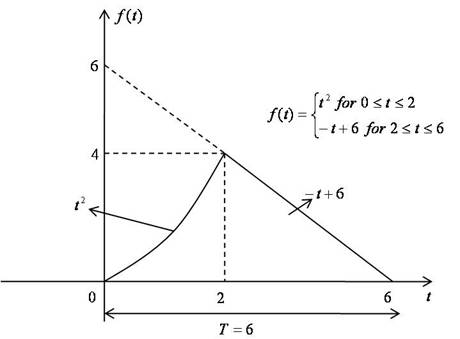# Quiz Chapter 11.02: Continuous Fourier Transforms

 MULTIPLE CHOICE TEST CONTINUOUS FOURIER TRANSFORMS FAST FOURIER TRANSFORMS

1. Which of the following is an even function of $t$?

2. A “periodic function” is given by a function which

3. Given the following periodic function, $f(t)$.The coefficient $a_{0}$ of the continuous Fourier series associated with the above given function $f(t)$ can be computed as

4. For the given periodic function

• $f(t) =\left\{\begin{matrix} 2t \,\, for \,\, 0 \leq t \leq 2 \\4 \,\, for \,\, 2 \leq t \leq 6\end{matrix}\right.$

with a period $T=6$. The coefficient $b_{1}$ of the continuous Fourier series associated with the given function $f(t)$ can be computed as

5. For the given periodic function

• $f(t) =\left\{\begin{matrix} 2t \,\, for \,\, 0 \leq t \leq 2 \\4 \,\, for \,\, 2 \leq t \leq 6\end{matrix}\right.$

with a period of $T=6$. The Fourier coefficient $a_{1}$ can be computed as

6. For the given periodic function

• $f(t) =\left\{\begin{matrix} 2t \,\, for \,\, 0 \leq t \leq 2 \\4 \,\, for \,\, 2 \leq t \leq 6\end{matrix}\right.$

with a period of $T=6$ as shown in Problem $5$. The complex form of the Fourier series  can be expressed as

• $f(t)=\displaystyle\sum_{k=-\infty}^{\infty}\widetilde{C}_{k} e^{ikw_{0}t}$

The complex coefficient $\widetilde{C}_{1}$ can be expressed as